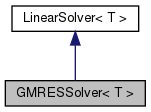Chombo + EB + MF  3.2
GMRESSolver< T > Class Template Reference

`#include <GMRESSolver.H>`

Inheritance diagram for GMRESSolver< T >:[legend]

## Public Member Functions

GMRESSolver ()

virtual ~GMRESSolver ()

void clearData ()

virtual void setHomogeneous (bool a_homogeneous)

virtual void setConvergenceMetrics (Real a_metric, Real a_tolerance)
Set a convergence metric, along with solver tolerance, if desired. More...

virtual void define (LinearOp< T > *a_op, bool a_homogeneous=true)

virtual void solve (T &a_phi, const T &a_rhs)
solve the equation. More...

int restartLen () const

void setRestartLen (int mm)Public Member Functions inherited from LinearSolver< T >
virtual ~LinearSolver ()

## Public Attributes

bool m_homogeneous

LinearOp< T > * m_op

int m_imax

int m_verbosity

Real m_eps

Real m_reps

int m_exitStatus

Real m_small

int m_normType

## Private Member Functions

void allocate ()

void CycleGMRES (T &xx, int &reason, int &itcount, Real &rnorm0, const bool avoidnorms=false)

void ResidualGMRES (T &a_vv, const T &a_xx, const T &a_bb, T &a_temp)

void BuildGMRESSoln (Real nrs[], T &a_xx, const int it, const T vv_0[])

void UpdateGMRESHessenberg (const int it, bool hapend, Real &res)

void TwoUnmodifiedGramSchmidtOrthogonalization (const int it)

void ApplyAB (T &a_dest, const T &a_xx, T &a_temp) const

int m_restrtLen

Realm_data

Realm_hes

Realm_hh

Realm_d

Realm_ee

Realm_dd

T * m_work_arr

## Detailed Description

### template<class T> class GMRESSolver< T >

Krylov solver using the GMRES algorithm. Important parmeters are: 1) relative tolerance: m_reps(1.e-12) 2) restart length: m_restrtLen(30)

## Constructor & Destructor Documentation

template<class T >
 GMRESSolver< T >::GMRESSolver ( )
template<class T >
 GMRESSolver< T >::~GMRESSolver ( )
virtual

## Member Function Documentation

template<class T >
 void GMRESSolver< T >::clearData ( )
template<class T >
 virtual void GMRESSolver< T >::setHomogeneous ( bool a_homogeneous )
inlinevirtual

reset whether the solver is homogeneous.

Implements LinearSolver< T >.

References GMRESSolver< T >::m_homogeneous.

template<class T >
 void GMRESSolver< T >::setConvergenceMetrics ( Real a_metric, Real a_tolerance )
virtual

Set a convergence metric, along with solver tolerance, if desired.

Default implementation does nothing, since there are probably cases (liked direct solves), where this has no real meaning.

Reimplemented from LinearSolver< T >.

template<class T >
 void GMRESSolver< T >::define ( LinearOp< T > * a_op, bool a_homogeneous = `true` )
virtual

define the solver. a_op is the linear operator. a_homogeneous is whether the solver uses homogeneous boundary conditions.

Implements LinearSolver< T >.

template<class T >
 void GMRESSolver< T >::solve ( T & a_phi, const T & a_rhs )
virtual

solve the equation.

m_exitStatus set = -1 if solver exited for an unknown reason set = 1 if solver converged to tolerance set = 2 if rho = 0 set = 3 if max number of restarts was reached

Implements LinearSolver< T >.

References CH_START, CH_STOP, CH_TIMER, CH_TIMERS, pout(), VEC_OFFSET, VEC_TEMP_LHS, VEC_TEMP_RHS, and VEC_VV.

template<class T >
 int GMRESSolver< T >::restartLen ( ) const
inline
template<class T >
 void GMRESSolver< T >::setRestartLen ( int mm )

References CH_assert.

template<class T >
 void GMRESSolver< T >::allocate ( )
private
template<class T >
 void GMRESSolver< T >::CycleGMRES ( T & xx, int & reason, int & itcount, Real & rnorm0, const bool avoidnorms = `false` )
private
template<class T >
 void GMRESSolver< T >::ResidualGMRES ( T & a_vv, const T & a_xx, const T & a_bb, T & a_temp )
private

References CH_assert.

template<class T >
 void GMRESSolver< T >::BuildGMRESSoln ( Real nrs[], T & a_xx, const int it, const T vv_0[] )
private

References GRS, HH, pout(), VEC_TEMP_LHS, and VEC_TEMP_RHS.

template<class T >
 void GMRESSolver< T >::UpdateGMRESHessenberg ( const int it, bool hapend, Real & res )
private

References Abs(), CC, GRS, HH, pout(), and SS.

template<class T >
 void GMRESSolver< T >::TwoUnmodifiedGramSchmidtOrthogonalization ( const int it )
private

References HES, HH, and VEC_VV.

template<class T >
 void GMRESSolver< T >::ApplyAB ( T & a_dest, const T & a_xx, T & a_temp ) const
private

## Member Data Documentation

template<class T >
 bool GMRESSolver< T >::m_homogeneous

public member data: whether the solver is restricted to homogeneous boundary conditions

Referenced by GMRESSolver< T >::setHomogeneous().

template<class T >
 LinearOp* GMRESSolver< T >::m_op

public member data: operator to solve.

template<class T >
 int GMRESSolver< T >::m_restrtLen
private

private member data: restart length, need to allocate stuff

Referenced by GMRESSolver< T >::restartLen().

template<class T >
 int GMRESSolver< T >::m_imax

public member data: max iterations (eg, >= m_restrtLen)

template<class T >
 int GMRESSolver< T >::m_verbosity

public member data: how much screen out put the user wants. set = 0 for no output.

template<class T >
 Real GMRESSolver< T >::m_eps

public member data: solver tolerance

template<class T >
 Real GMRESSolver< T >::m_reps

public member data: relative solver tolerance

template<class T >
 int GMRESSolver< T >::m_exitStatus

public member data: set = -1 if solver exited for an unknown reason set = 1 if solver converged to tolerance set = 2 if rho = 0 set = 3 if max number of restarts was reached

template<class T >
 Real GMRESSolver< T >::m_small

public member data: what the algorithm should consider "close to zero"

template<class T >
 int GMRESSolver< T >::m_normType
template<class T >
 Real* GMRESSolver< T >::m_data
private
template<class T >
 Real* GMRESSolver< T >::m_hes
private
template<class T >
 Real * GMRESSolver< T >::m_hh
private
template<class T >
 Real * GMRESSolver< T >::m_d
private
template<class T >
 Real * GMRESSolver< T >::m_ee
private
template<class T >
 Real * GMRESSolver< T >::m_dd
private
template<class T >
 T* GMRESSolver< T >::m_work_arr
private

The documentation for this class was generated from the following file: